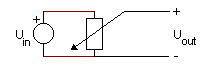﻿ 20-sim webhelp > Library > Iconic Diagrams > Mechanical > Rotation > Sensors > potentiometer

# potentiometer

## Library

Iconic Diagrams\Mechanical\Rotation\Sensors

## Use

Domains: Continuous. Size: 1-D. Kind: Iconic Diagrams (Rotation / Electric).

## Description

Potentiometers have a variable resistance according to a certain rotation. By establishing a voltage (see picture below) across the resistor it is possible to get a proportional relation between the angle and the output voltage.with

Uout = Umax * angle / (turns*2*pi)

Here Umax is the maximum voltage and turns is the maximum number of turns.

## Interface

Ports

Description

p1

p2

Rotation port.

Electric port.

Causality

fixed torque out p1

fixed voltage out p2

(The output torque is zero)

The output is equal to Uout.

## Parameters

Umax

turns

Maximum output voltage [V]

maximum allowed number of turns []